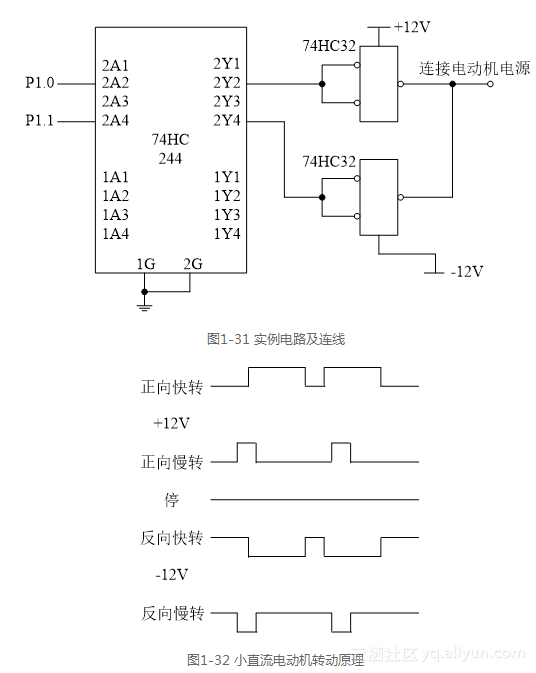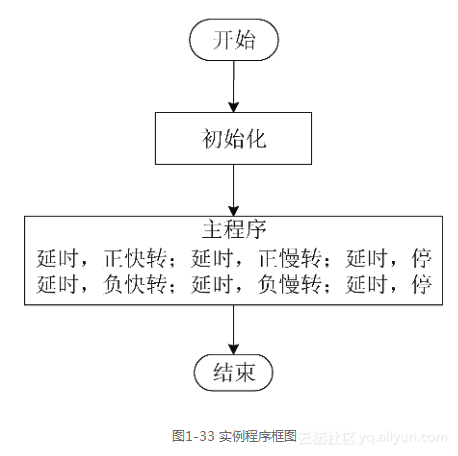# 《51单片机应用开发范例大全(第3版)》——1.4 【实例19】P1口控制直流电动机实例

+关注继续查看

### 1.4 【实例19】P1口控制直流电动机实例

1．实例概述2．程序框图及代码sfr p1=0x90;
sbit p10=p1^0;
sbit p11=p1^1;

void main ()
{
int i, m;
int j=100;
int k=20;

// 正快转
for (i=0; i<100; i++)
{
P10=1;
for (j=0; j<50; j++)
{
m=0;
}
}
P10=0;
for (j=0; j<10; j++)
{
m=0
}
//正慢转
for (i=0; i<100; i++)
{
P10=1;
for (j=0; j<10; j++)
{
m=0
}
}
p10=0;
for (j=0; j<50; j++)
{
m=0
}
// 负快转
for (i=0; i<100; i++)
{
p11=1;
for (j=0; j<50; j++)
{
m=0;
}
}
p11=0;
for (j=0; j<10; j++)
{
m=0;
}

// 负慢转
for (i=0; i<100; i++)
{
p11=1;
for (j=0;j<10;j++)
{
m=0;
}
}
p11=0
for (j=0; j<50; j++)
{
m=0;
}
}
Cx51源程序是一个ASCII文件，可以用任何标准的ASCII文本编辑器来编写，例如记事本、写字板等。

C源程序的书写格式自由度较高，灵活性很强，有较大的任意性。其要点如下。

11938

0

《SaaS模式云原生数据仓库应用场景实践》

《看见新力量：二》电子书